1603054800

Text Classification Using Naive Bayes: Theory & A Working Example

1. Introduction
2. The Naive Bayes algorithm
3. Dealing with text data
4. Working Example in Python (step-by-step guide)
5. Bonus: Having fun with the model
6. Conclusions

1. Introduction

Naive Bayes classifiers are a collection of classification algorithms based on Bayes’ Theorem. It is not a single algorithm but a family of algorithms where all of them share a common principle, i.e. every pair of features being classified is independent of each other.

Naive Bayes classifiers have been heavily used for text classification and text analysis machine learning problems.

Text Analysis is a major application field for machine learning algorithms. However the raw data, a sequence of symbols (i.e. strings) cannot be fed directly to the algorithms themselves as most of them expect numerical feature vectors with a fixed size rather than the raw text documents with variable length.

In this article I explain a) how **Naive Bayes works, **b) how we can use text data and fit them into a model after transforming them into a more appropriate form. Finally, I implement a multi-class text classification problem step-by-step in Python.

Let’s get started !!!

#probability #classification #naive-bayes #data-science #machine-learning

Buddha Community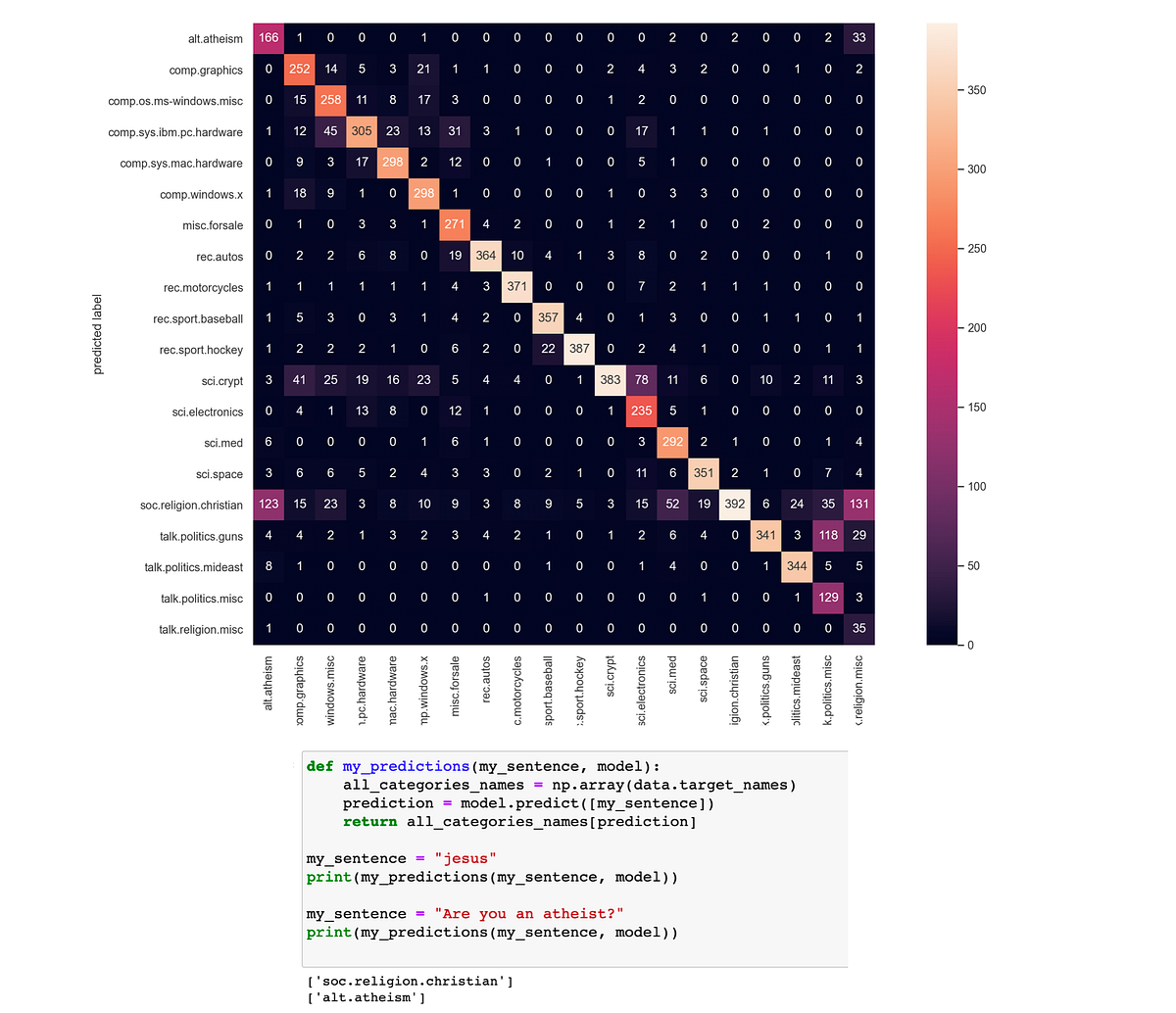1603054800

Text Classification Using Naive Bayes: Theory & A Working Example

1. Introduction
2. The Naive Bayes algorithm
3. Dealing with text data
4. Working Example in Python (step-by-step guide)
5. Bonus: Having fun with the model
6. Conclusions

1. Introduction

Naive Bayes classifiers are a collection of classification algorithms based on Bayes’ Theorem. It is not a single algorithm but a family of algorithms where all of them share a common principle, i.e. every pair of features being classified is independent of each other.

Naive Bayes classifiers have been heavily used for text classification and text analysis machine learning problems.

Text Analysis is a major application field for machine learning algorithms. However the raw data, a sequence of symbols (i.e. strings) cannot be fed directly to the algorithms themselves as most of them expect numerical feature vectors with a fixed size rather than the raw text documents with variable length.

In this article I explain a) how **Naive Bayes works, **b) how we can use text data and fit them into a model after transforming them into a more appropriate form. Finally, I implement a multi-class text classification problem step-by-step in Python.

Let’s get started !!!

#probability #classification #naive-bayes #data-science #machine-learning1596465840

The Ironic Sophistication of Naive Bayes Classifiers

Filtering spam with Multinomial Naive Bayes (From Scratch)

In the first half of 2020 more than 50% of all email traffic on the planet was spam. Spammers typically receive 1 reply for every 12,500,000 emails sent which doesn’t sound like much until you realize more than 15 billion spam emails are being sent each and every day. Spam is costing businesses 20–200 billion dollars per year and that number is only expected to grow.What can we do to save ourselves from spam???

Naive Bayes Classifiers

In probability theory and statistics, Bayes’ theorem (alternatively Bayes’s theoremBayes’s law or Bayes’s rule) describes the probability of an event, based on prior knowledge of conditions that might be related to the event.

For example, if the risk of developing health problems is known to increase with age, Bayes’s theorem allows the risk to an individual of a known age to be assessed more accurately than simply assuming that the individual is typical of the population as a whole.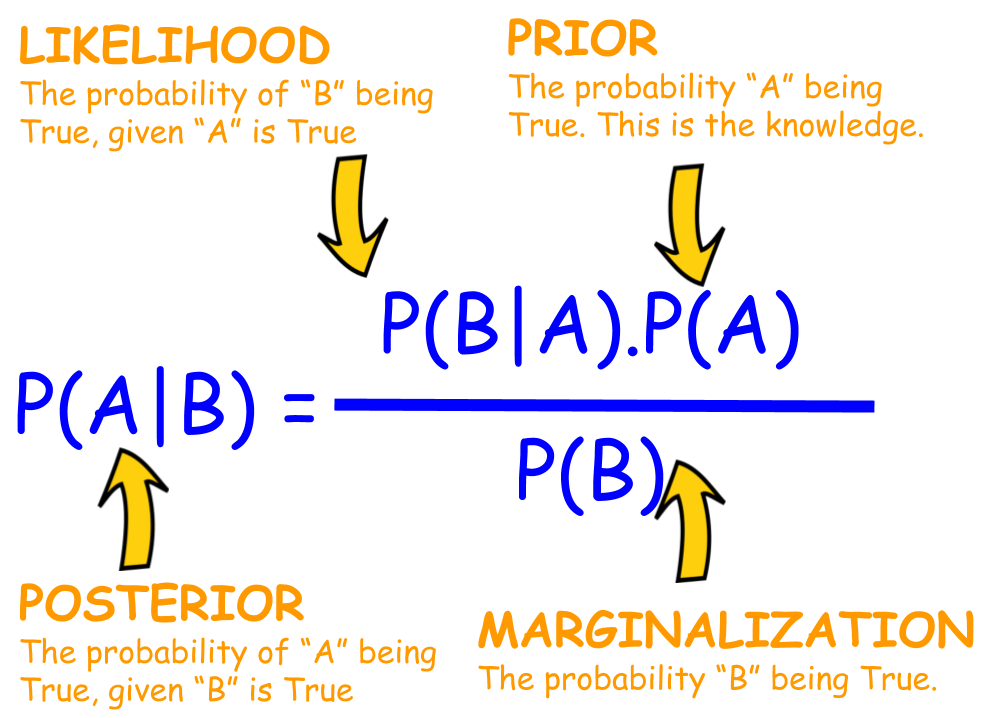Bayes Theorem Explained

A Naive Bayes Classifier is a probabilistic classifier that uses Bayes theorem with strong independence (naive) assumptions between features.

• Probabilistic classifier: a classifier that is able to predict, given an observation of an input, a probability distribution over a set of classes, rather than only outputting the most likely class that the observation should belong to.
• Independence: Two events are **independent **if the occurrence of one does not affect the probability of occurrence of the other (equivalently, does not affect the odds). That assumption of independence of features is what makes Naive Bayes naive! In real world, the independence assumption is often violated, but naive Bayes classiﬁers still tend to perform very well.

#naive-bayes-classifier #python #naive-bayes #naive-bayes-from-scratch #naive-bayes-in-python1599007020

Bayes Theorem and Text Classification using Naive Bayes Classifier

This article discusses Bayes theorem and how Naive Bayes classifier is used in text classification.

Firstly, We will consider a problem of finding the probability of fire given smoke assuming we were given certain information_. _In order to get to _p(Fire| Smoke) _or p(Fire given Smoke), let us draw an sample space where we can see all possibilities, as below:

To find p(Fire| Smoke), we are only concerned with areas where Smoke(Evidence) is present {1} and {3} as we are constrained to our evidence GIVEN smoke, so p(Fire| Smoke) will be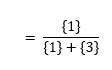or we can also write it has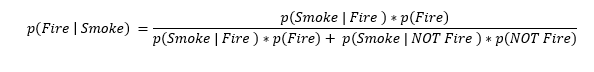Generalizing it for some Event given Evidence: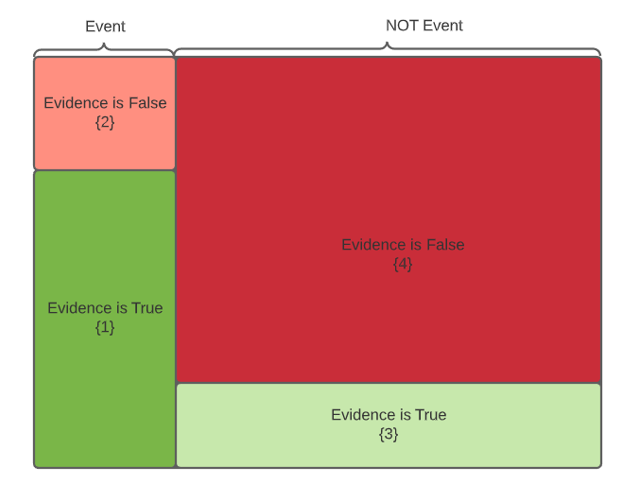As discussed, we have two areas of consideration 1. Evidence True- Event Happening Space {1} . 2. Evidence True- Event NOT happening space {3}.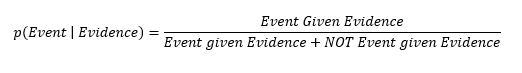(or)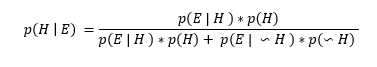Same formula can be re-written as below, basically in denominator we are adding up all the areas where Evidence is True.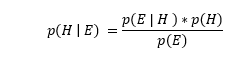We have arrived at Bayes theorem formula, basically Bayes rule describes the probability of an event in the light of evidence. More generally, finding the probability of an event based on prior knowledge can be written as: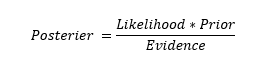#text-classification #naive-bayes #machine-learning #ebay1594262076

How to Build a Spam Filter using Python and Naive Bayes

In this blog post, we’re going to build a spam filter using Python and the multinomial Naive Bayes algorithm. Our goal is to code a spam filter from scratch that classifies messages with an accuracy greater than 80%.

To build our spam filter, we’ll use a dataset of 5,572 SMS messages. Tiago A. Almeida and José María Gómez Hidalgo put together the dataset, you can download it from the UCI Machine Learning Repository.

We’re going to focus on the Python implementation throughout the post, so we’ll assume that you are already familiar with multinomial Naive Bayes and conditional proability.

If you need to fill in any gaps before moving forward, Dataquest has a course that covers both conditional probability and multinomial Naive Bayes, as well as a broad variety of other course you could use to fill in gaps in your knowledge and earn a data science certificate.

Exploring the Dataset

Let’s start by opening the SMSSpamCollection file with the read_csv() function from the pandas package. We’re going to use:

• sep='\t' because the data points are tab separated
• names=['Label', 'SMS'] to name the columns

#classification #naive bayes #python #text classification1598404620

Hands-on Guide to Pattern - A Python Tool for Effective Text Processing and Data Mining

Text Processing mainly requires Natural Language Processing( NLP), which is processing the data in a useful way so that the machine can understand the Human Language with the help of an application or product. Using NLP we can derive some information from the textual data such as sentiment, polarity, etc. which are useful in creating text processing based applications.

Python provides different open-source libraries or modules which are built on top of NLTK and helps in text processing using NLP functions. Different libraries have different functionalities that are used on data to gain meaningful results. One such Library is Pattern.

Pattern is an open-source python library and performs different NLP tasks. It is mostly used for text processing due to various functionalities it provides. Other than text processing Pattern is used for Data Mining i.e we can extract data from various sources such as Twitter, Google, etc. using the data mining functions provided by Pattern.